Included chapter-wise all formulas with tips of Practice Maths better## Complete List of All Class 10 Maths Formulas: Chapterwise PDF Download

Maths is a subject that most students do not like because they don’t understand it well and more likely feel connected with it. Especially the class 10 maths formulas, the reason behind this is simple: students' base concepts are not solid. Having a solid base is required for everything to build perfectly.

Let’s take an example: when we build our house, we make the base should be solid so that house will stay intact in the storm also. The same rule applies to maths also. If we knew all the formulas and techniques to calculate the answer then solving answers would become easy.

Scoring good marks in maths is not tough. Although, in maths we get answers as per the right steps will calculate. So to increase your maths marks and understanding as well , read this article till last and you will get all your answers. We make it easy for all students to learn maths easily.

### General Class 10 Maths Formulas

These are formulas which are required in every other chapter. So these are must learn formulas. Check the complete list of class 10 general formulas below, these are helpful to solve most of the maths questions.

1. (a+b)2 = a2 + b2 + 2ab
2. (a-b)2 = a2 + b2 – 2ab
3. (a+b) (a-b) = a2 – b2
4. (x + a)(x + b) = x2 + (a + b)x + ab
5. (x + a)(x – b) = x2 + (a – b)x – ab
6. (a + b)3 = a3 + b3 + 3ab(a + b)
7. (a – b)3 = a3 – b3 – 3ab(a – b)
8. (x – a)(x + b) = x2 + (b – a)x – ab
9. (x – a)(x – b) = x2 – (a + b)x + ab
10. (x + y + z)2 = x2 + y2 + z2 + 2xy + 2yz + 2xz
11. (x + y – z)2 = x2 + y2 + z2 + 2xy – 2yz – 2xz
12. (x – y + z)2 = x2 + y2 + z2 – 2xy – 2yz + 2xz
13. (x – y – z)2 = x2 + y2 + z2 – 2xy + 2yz – 2xz
14. x3 + y3 + z3 – 3xyz = (x + y + z)(x2 + y2 + z2 – xy – yz -xz)
15. x2 + y2 =½ [(x + y)2 + (x – y)2]
16. (x + a) (x + b) (x + c) = x3 + (a + b +c)x2 + (ab + bc + ca)x + abc
17. x3 + y3= (x + y) (x2 – xy + y2)
18. x3 – y3 = (x – y) (x2 + xy + y2)
19. x2 + y2 + z2 -xy – yz – zx = ½ [(x-y)2 + (y-z)2 + (z-x)2]

## Class 10 Maths Formulas for All Chapters

Check all the chapter-wise formulas of class 10 maths. Students do not have to check any other article after reading this because you will get all the formulas in table and pdf form in the one place. Check all formulas below:

### Class 10 Maths Chapter 1 Real Numbers Formulas

Chapter 1 real numbers mostly consist of very few formulas. Check all the formulas in the table given below.

Natural Numbers N ={ 1, 2,3,4,5 … }
Whole Numbers W={ 0, 1, 2, 3, 4, 5… }
Rational Numbers Those numbers which can be presented in the form of a/b are called Rational Numbers.
Re Numbers Real Numbers can be found on a number line

### Class 10 Maths Chapter 3 Pair of Linear Equations in Two Variables Formulas

All the formulas of chapter 3 pair of linear equations in two variables are given below.

1. Linear equation in one variable: ax +b =0
2. Linear equation in two variables: ax+ by+ c =0
3. Linear equation in three variables: ax+ by+ cz= 0

### Class 10 Maths Chapter 5 Arithmetic Progression Formulas

Terms of sequence are denoted by a1 a2, a3, …………… an. An arithmetic progression is a sequence of numbers such that the difference between the consecutive terms is equal.

1. an = a + (n - 1) d, where an is the nth term.
2. Sn= n/2 [2a + (n - 1)d]

### Class 10 Maths Chapter 8 Trigonometry Formulas

Class 10 chapter 8 trigonometry basically covers three parts sine, cosine, and tangent and the opposite of these are sec, cosec and cot. Below are all the formulas used in trigonometry chapter.

Sin θ = Side opposite to angle θ/ Hypotenuse = Perpendicular/Hypotenuse = P/H

Cos θ = Adjacent side to angle θ/ Hypotenuse = Base/ Hypotenuse = B/H

Tan θ = Side opposite to angle θ/ Adjacent side to angle θ = P/B

Cosec θ = 1/ sin θ

Sec θ = 1/ cos θ

Cot = 1/ tan θ

Tan θ = sin θ/ cos θ

#### Trigonometry Table for Class 10 Chapter 8 Trigonometry

θ 30° 45° 60° 90° 180°
Sin 0 1/2 1/2 3/2 1 0
Cos 1 3/2 1/2 1/2 0 -1
Tan 0 1/3 1 3 0
Cot 3 1 1/3 0
Sec 1 2/3 2 2 -1
Cosec 2 2 2/3 1

• Sinθ= 1/ Cosec θ or Sinθ.Cosθ= 1
• Cosθ= 1/Secθ or Cos θ.Secθ= 1
• Tanθ= 1/Cotθ or Tanθ.Cotθ= 1
• Sin (A+B)= SinA.CosB + CosA.SinB
• Sin (A-B)= SinA.CosB – CosA.SinB
• Cos (A+B)= CosA.CosB- SinA.SinB
• Cos (A-B)= CosA.CosB+SinA.SinB
• Tan (A+B)= (TanA + TanB)/ (1-TanA TanB)
• Tan (A-B)= (TanA- TanB)/ (1+TanA TanB)

### Additional Maths Formulas for Class 10 Trigonometry

Other than chapter formulas, below are additional formulas which will be commonly used in all the questions of class 10 chapter 8 trigonometry. All the formulas are given below.

1. sin θ = Side opposite to angle θ/ Hypotenuse = Perpendicular/Hypotenuse= P/H
2. cos θ = Adjacent side to angle θ/ Hypotenuse= Adjacent Side/ Hypotenuse= B/H
3. tan θ = Side opposite to angle θ/ Adjacent side to angle θ
4. sec θ = 1/ cosθ
5. cot θ = 1/ tanθ
6. cosec θ = 1/ sinθ
7. tan θ = Sinθ/ Cosθ
8. sin (90° – θ) = cos θ
9. cos (90° – θ) = sin θ
10. tan (90° – θ) = cot θ
11. cot (90° – θ) = tan θ
12. sec (90° – θ) = cosecθ
13. cosec (90° – θ) = secθ
14. sin2θ + cos2 θ = 1
15. sec2 θ = 1 + tan2θ for 0° ≤ θ < 90°
16. Cosec2 θ = 1 + cot2 θ for 0° ≤ θ ≤ 90°

### Class 10 Maths Chapter 10 Area of Circle Formulas

All the formulas of chapter 10 area of circle have these two formulas which are given below.

1. The tangent to a circle equation x2 + y2 = a2 for a line y = mx + c is given by the equation y = mx ± a √[1+ m2].
2. The tangent to a circle equation x2 + y2 = a2 at (a1,b1) is xa1 + yb1 = a2

### Class 10 Maths Chapter 13 Surface Area and Volume Formulas

The common formulas of chapter 13 surface area and volume are given in the table below.

Sphere
Volume of Sphere 4/3 ×π r3
Lateral Surface Area of Sphere (LSA) 4π r2
Total Surface Area of Sphere (TSA) 4πr2
Right Circular Cylinder
Volume of Right Circular Cylinder πr2h
Lateral Surface Area of Right Circular Cylinder (LSA) 2×(πrh)
Total Surface Area of Right Circular Cylinder (TSA) 2πr×(r + h)
Hemisphere
Volume of Hemisphere ⅔ x (πr3)
Lateral Surface Area of Hemisphere (LSA) 2πr2
Total Surface Area of Hemisphere (TSA) 3πr2
Prism
Volume of Prism B × h
Lateral Surface Area of Prism (LSA) p × h

### Class 10 Maths Chapter 14 Statistics Formulas

Chapter 14 Statistics mostly deal with finding mean, mode, median. Check all the statistics formulas in the table below.

Mean of Grouped Data
Mean Direct Method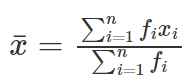where ∑fi xi is the sum of observations from value i = 1 to n
And ∑fi is the number of observations from value i = 1 to n
Assumed Mean Method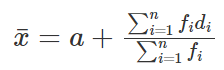where a is assumed mean and di is deviation of a from each of the xi.
Also, di = xi – a
Stop Deviation Method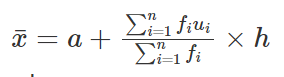Where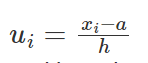and h is class size
Mode of Grouped Data
Mode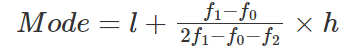l=lower limit of modal class.
h= size of the class interval
f1= frequency of modal class.
f0= frequency of the class preceding the modal class.
f2= frequency of the class succeeding the modal class.
Median of Grouped Data
Median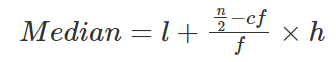l=lower limit of median class.
n= number of observations.
cf= cumulative frequency of class preceding the median class.
f= frequency of median class
h= class size

### Class 10 Maths Chapter 15 Probability Formulas

The essential formula for the Probability chapter is:

P(E)= Number of outcome favourable/ Number of all possible outcomes of the experiment

### All Class 10 Maths Formula PDF

Here is a complete pdf of all maths formulas, download through the button given below.

### Why You Need Strategy to Remember Maths Formulas

We check class 10 maths formulas of maths and now it's time to remember them. But you must be asked why you need tips to remember the formulas.

The maths formulas are a way to understand maths concepts in a concise way. They allow us to solve questions quickly and efficiently. Here some formulas are easy to remember and some are tough.

Therefore, having a right way to remember them is required. Learning formulas and practising them make our critical-thinking and problem solving skills better. Our overall performance improves.

### Tips to Memorise Class 10 Maths Formulas

Here are some tips to understand and remember class 10 all formulas of maths is an efficient way. Tips to remember formulas will definitely help in the long-term.

#### Tip 1: Create Mnemonics

Mnemonics are a memory aid which are made of combinations such as words, letters, numbers and images. It helps in recalling formulas faster. Check the different ways below to see how you can make your own mnemonics.

Here are the four ways to remember mnemonics better:

#### 1) Acronyms

Pick the first letter of each word in the formulas to create a new word or phrase to easily remember the formulas. For example: the acronym for Pi (π) to 7 decimal places will be “May I have a large container of coffee?”. To recall this count the letters in each word – 3.1415926.

#### 2) Rhymes

Create a rhyme or jingle that incorporates the different parts of the formula. For example: Pi (π) to 30 decimal places. The value of 3.1415926535897932384626433832795. Rhymes for this will be:

Now I, even I, would celebrate

In rhymes unapt, the great

Immortal Syracusan, rivaled nevermore,

Who in his wondrous lore,

Passed on before,

Left men his guidance

How to menstruate circles.

By using the mnemonics it becomes easier to recall formulas, which directly helps us to solve problems and make our calculation more efficient.

#### Tip 2: Break It Down

Break down will help to understand the components of the formula and how they relate to one another. Once you break down the formula in a meaningful way then it becomes easier to remember and recall it. Identifying the variables and practice will make things smooth and help to get the final result.

#### Tip 3: Repetition

Repetition is a powerful way to learn formulas faster. The more you repeat a formula, the more likely you are to remember it. Repeat the formula multiple times until it becomes second nature.

These are some ways of repetition: quiz, flashcards, daily repetition, repeating formulas in different formats and daily practice. By repeating the formula regularly, it becomes more familiar and easier to recall in the long-term.

### Conclusion

All the class 10 maths formulas are added above, students do not have to go anywhere in the search of formulas. Practise all these formulas to make your preparation better. When your preparation is strong, then you can solve questions quickly and accurately, and it will also help you to understand advanced level questions in maths.